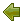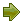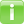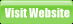Project
Code
Meter

## Maintainability Index (MI)

[article cited from Wikipedia]

Maintainability Index is a software metric which measures how maintainable (easy to support and change) the source code is. The maintainability index is calculated as a factored formula consisting of Lines Of Code, Cyclomatic Complexity and Halstead volume. It is used in several automated software metric tools, including the Microsoft Visual Studio 2010 development environment, which uses a shifted scale (0 to 100) derivative.

### Calculation

First we need to measure the following metrics from the source code:

• G = Cyclomatic Complexity
• LOC = count of source Lines Of Code (SLOC)
• CM = percent of lines of Comment (optional)

From these measurements the MI can be calculated:

The original formula:
MI = 171 - 5.2 * ln(V) - 0.23 * (G) - 16.2 * ln(LOC)

The derivative used by SEI is calculated as follows:
MI  = 171 - 5.2 * log2(V) - 0.23 * G - 16.2 * log2 (LOC) + 50 * sin (sqrt(2.4 * CM))

The derivative used by Microsoft Visual Studio (since v2008) is calculated as follows:
MI = MAX(0,(171 - 5.2 * ln(Halstead Volume) - 0.23 * (Cyclomatic Complexity) - 16.2 * ln(Lines of Code))*100 / 171)

In all derivatives of the formula, the most major factor in MI is Lines Of Code, which effectiveness have been subjected to debate.